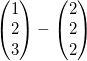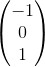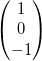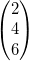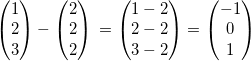# Precalculus : Matrices and Vectors

## Example Questions

### Example Question #161 : Matrices And Vectors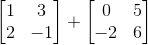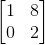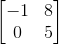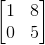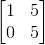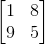Explanation:

To add two matrices, combine the corresponding numbers in the matrices: (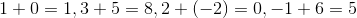). Then, put those answers in the corresponding spots in the answer matrix. Therefore, your answer is.

### Example Question #832 : Pre Calculus

Find the sum of the two matrices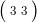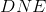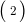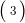Explanation:

Because the matrices do not have the same dimension, the sum does not exist.

### Example Question #1 : Find The Difference Of Two Matrices

Subtract: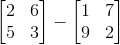Explanation:

In order to subtract matrices, they have to be of the same dimension. In this case, they are both 2x2.

The formula to subtract matrices is as follows: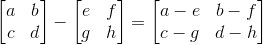Using this formula we plug in our values and solve: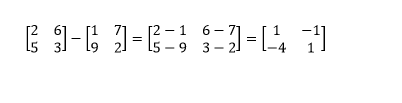### Example Question #2 : Find The Difference Of Two Matrices

Subtract: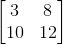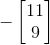No SolutionNo Solution

Explanation:

In order to subtract matrices, they have to be of the same dimension. In this case, they are not. One is 2x2 while the other is 2x1.

As such, we cannot find their difference.

The answer is No Solution

### Example Question #3 : Find The Difference Of Two Matrices

Subtract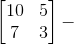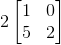Explanation:

The first step in solving this problem is to multiply the matrix by the scalar.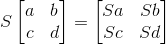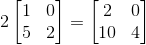We then have to make sure we can subtract the matrices.

In order to subtract matrices, they have to be of the same dimension. In this case, they are both 2x2.

Using our new matrix from multiplying the scalar and subtracting it from our other matrix we get the following: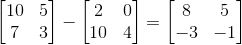### Example Question #4 : Find The Difference Of Two Matrices

We consider the following matrices: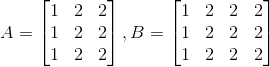Find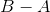.We can't find.We can't find.

Explanation:

To be able to subtract matrices, the matrices must have the same size.

A is 3x3 and B is 3x4. Therefore we can't perform this operation.

### Example Question #1 : Matrices

Given the following matrices, what is the product ofand?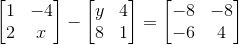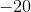Explanation:

When subtracting matrices, you want to subtract each corresponding cell.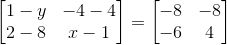Now solve forand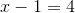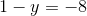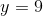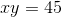### Example Question #1 : Find The Difference Of Two Matrices

If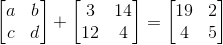, what is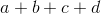?Explanation:

You can treat matrices just like you treat other members of an equation. Therefore, you can subtract the matrix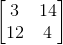from both sides of the equation.  This gives you: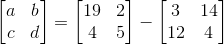Now, matrix subtraction is simple. You merely subtract each element, matching the correlative spaces with each other: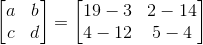Then, you simplify: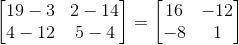Therefore,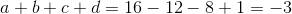### Example Question #7 : Find The Difference Of Two Matrices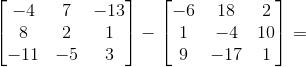Explanation:

To find the difference between two matrices with the same dimensions, we simply subtract each entry from the right matrix from the corresponding entry of the left matrix. After performing the operation 9 times, we get the following matrix: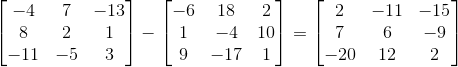### Example Question #1 : Find The Difference Of Two Matrices

Find the difference if it exists